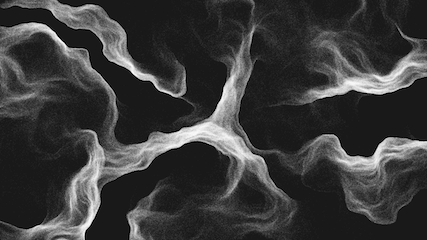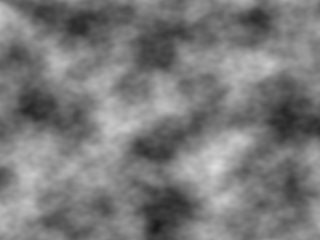In many of the programs we build with p5.js, from video games to generative art to interactive applications, we sometimes like to have a bit of randomness to create unexpected and dynamic behavior. Many times though, the random() function is too random -- sometimes we want a function that wanders around but remains smooth and organic.

For this reason, p5.js has a function called noise(), which generates these smooth gradients using a function called Perlin noise, named after its inventor, Ken Perlin. Perlin noise was invented for applications which required semi-random variations with continuous and smooth trajectories. It's used in all sorts of applications, such as creating terrains and realistic looking textures for video games. The following pictures show some uses of noise.The noise() function in p5.js takes 1, 2, or 3 arguments. Let's start by looking at noise with 1 argument. Take a look at the following example.

``` function setup() { createCanvas(400, 400); } function draw() { background(0); noFill(); stroke(255); beginShape(); for (var x = 0; x < width; x++) { var nx = map(x, 0, width, 0, 10); var y = height * noise(nx); vertex(x, y); } endShape(); } ```

In the above sketch, we are plotting y=noise(nx) between nx=0 and nx=10. noise(x) always gives us back a number between 0 and 1 -- in this example, we scale it up by multiplying it by the height of the canvas, so the result is between 0 and height. There are two key characteristics to observe. The first is that unlike random(), noise() always gives us back the same value for the same arguments. So noise(5) will give us some value when we run it at some point during our sketch, and if we run noise(5) again later in the sketch, we will get the same value. The second important thing is that we expect to get closer y-values for closer x-values inputted to noise(). So for example, noise(5) and noise(10) may give very different values, but noise(5) and noise(5.5) will probably be comparatively closer to each other, and noise(5) and noise(5.001) will be very close to each other.

This last property is the key that lets us create and control very interesting trajectories -- by riding along a narrow band of noise, we get smooth continuous movement along an overall random path. By riding a larger band, we get faster and more varied movement. Try changing the range of the argument to noise (nx). What happens if instead of going from 0 to 10, we go from 0 to 100 instead? Or 0 to 1? Notice the smaller the range of values we traverse, the more smooth our movement becomes.

To see this in more detail, see the sketch below. Here we take the value of the noise function and map it to the y-position of a circle. At the same time, we increment the noise function by an amount which is controlled by the x-position of the mouse. As you move the mouse to the right, we increment the noise argument by larger amounts. By stepping through the argument in noise at increasingly larger amounts, the output becomes increasingly more erratic. Eventually, it is almost indistinguishable from pure randomness. Stepping through the noise field by very small amounts gives us very slow and smooth movement.

``` var t; var t_increment; function setup() { createCanvas(400, 400); textSize(16); t = 0; t_increment = 0; } function draw() { background(0); // t_increment will control how "fast" we move // through the 1d noise graph t_increment = map(mouseX, 0, width, 0, 0.2); var y = height * noise(t); // add t_increment to t t += t_increment; fill(0, 255, 0); ellipse(width/2, y, 60, 60); fill(255); text("t_increment = "+t_increment, 20, 30); text("t = "+t, 20, 60); text("noise(t) = "+noise(t), 20, 90); text("height * noise(t) = "+height * noise(t), 20, 120); } ```

So what can we do with Perlin noise? One thing is we can create variables which move in seemingly random but organic ways. In the following sketch, we draw a simple circle whose position and color change along a smooth noise field.

``` var t; function setup() { createCanvas(400, 400); background(0); t = 0; } function draw() { // fade the background by giving it a low opacity background(0, 5); var x = width * noise(t); var y = height * noise(t+5); var r = 255 * noise(t+10); var g = 255 * noise(t+15); var b = 255 * noise(t+20); noStroke(); fill(r, g, b); ellipse(x, y, 120, 120); t = t + 0.01; } ```

Wait... why did we add a constant number (5, 10, etc) to the t variable in each noise function? Recall that noise(x) always gives you the same output for any value of x, no matter how many times you call it in the same sketch. If we had simply used noise(t) for each of our five variables x, y, r, g, and b, they would have remained equal to each other at each point t -- thus we'd always have a gray circle moving along the diagonal line y=x between (0, 0) and (width, height)! Try removing that constant and see that it does that. By leaving that constant there as an offset, we ensure all of the values are different by moving them along different "regions" of the noise field. There is a better way to do this though, as we will see later...

As with the previous sketches, the smoothness of our movement depends on how fast we step through the noise field. In this sketch, that is controlled by the last line, t = t + 0.01. What happens if we increment it by a value more than or less than 0.01? Or even better, what if the increment value is itself controlled by a noise function! Try experimenting with this to get more intuition about how the noise function works.

Here's a more interesting example of noise in action. Here we are continuously drawing a single bezier curve to the canvas, whose end points and control points are all being moved according to noise. Notice again we use a small offset to make sure the noise values are different for each of the 8 variables. Accumulating these beziers on the canvas creates very interesting contours, giving it an almost 3d feel.

``` var t; function setup() { createCanvas(400, 400); stroke(0, 18); noFill(); t = 0; } function draw() { var x1 = width * noise(t + 15); var x2 = width * noise(t + 25); var x3 = width * noise(t + 35); var x4 = width * noise(t + 45); var y1 = height * noise(t + 55); var y2 = height * noise(t + 65); var y3 = height * noise(t + 75); var y4 = height * noise(t + 85); bezier(x1, y1, x2, y2, x3, y3, x4, y4); t += 0.005; // clear the background every 500 frames using mod (%) operator if (frameCount % 500 == 0) { background(255); } } ```

# 2D and 3D Perlin noise

A lot of times we need smooth continuous behavior from a variable in not just one but two dimensions. For example, if you are rendering clouds, you might want to have smooth color gradations in both the X and Y directions. For this reason, the noise() function can take two arguments instead of one, where the second argument works exactly like the first one. Take a look at the following example.

``` function setup() { createCanvas(400, 400); noStroke(); } function draw() { for (var x = 0; x < width; x+=10) { for (var y = 0; y < height; y+=10) { var c = 255 * noise(0.01 * x, 0.01 * y); fill(c); rect(x, y, 10, 10); } } } ```

In the above example, we see that smooth gradations are visible in both dimensions. But having a second dimension to noise can be useful for more than just the X and Y directions; it can also be used for time-varying behavior as well. Take a look at the next, somewhat more complex, example. Here we are manually drawing a "circle" point by point, and as we go around, we deform the radius using the noise function, where one of the arguments is the angle from the center point. At the same time, the noise function is also varying in time. Thus each point in the circle is varied according to both time and angle, accumulating a series of deformed circles over time. For clarity, we clear the background every 600 frames.

``` var t; function setup() { createCanvas(400, 400); background(255); stroke(0, 15); noFill(); t = 0; } function draw() { translate(width/2, height/2); beginShape(); for (var i = 0; i < 200; i++) { var ang = map(i, 0, 200, 0, TWO_PI); var rad = 200 * noise(i * 0.01, t * 0.005); var x = rad * cos(ang); var y = rad * sin(ang); curveVertex(x, y); } endShape(CLOSE); t += 1; // clear the background every 600 frames using mod (%) operator if (frameCount % 600 == 0) { background(255); } } ```

The noise function can even take three arguments! So you can make it vary according to up to three different variables. This can be useful for example if you're creating a 3d scene, and you want a color value (or some other variable) to vary smoothly in all three spatial directions. Or another use is to add time-varying behavior to 2D Perlin noise. What if in the first 2D noise example with the rectangles, we added a third dimension to the noise variable controlling the darkness of the rectangles? That one is left up to you as an exercise!

# Recap

In this tutorial, we learned how to use the noise function to create smooth organically-varying variables in p5.js. Perlin noise enables us to exert some control over the randomness of our variables, and is widely used for creating realistic textures, generative art, AI motions, and many more applications. Having an understanding of how to master it will give you a great way of adding dynamic behaviors to your programs.